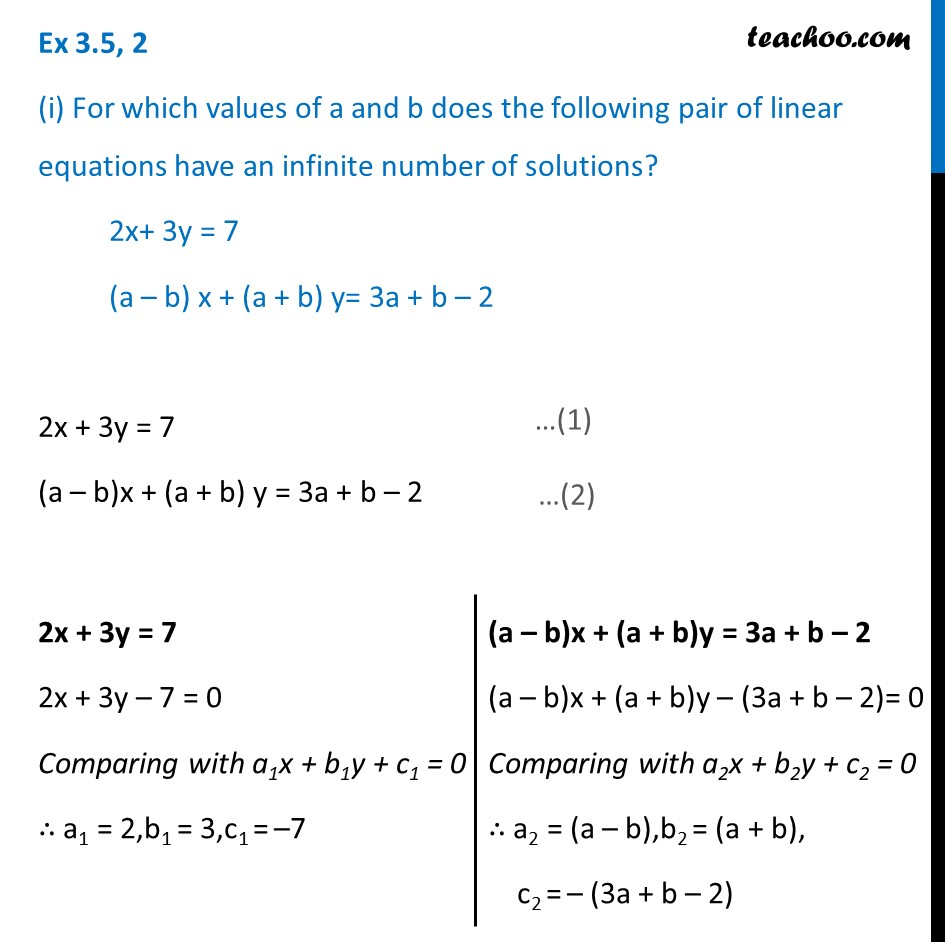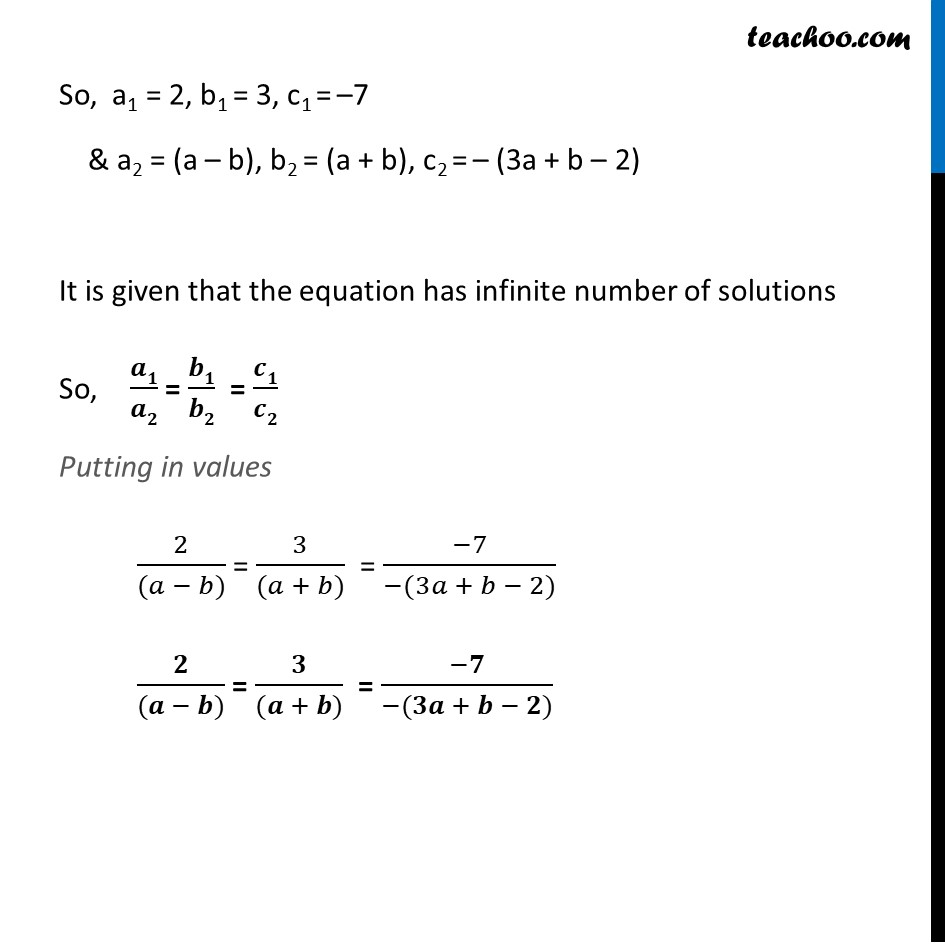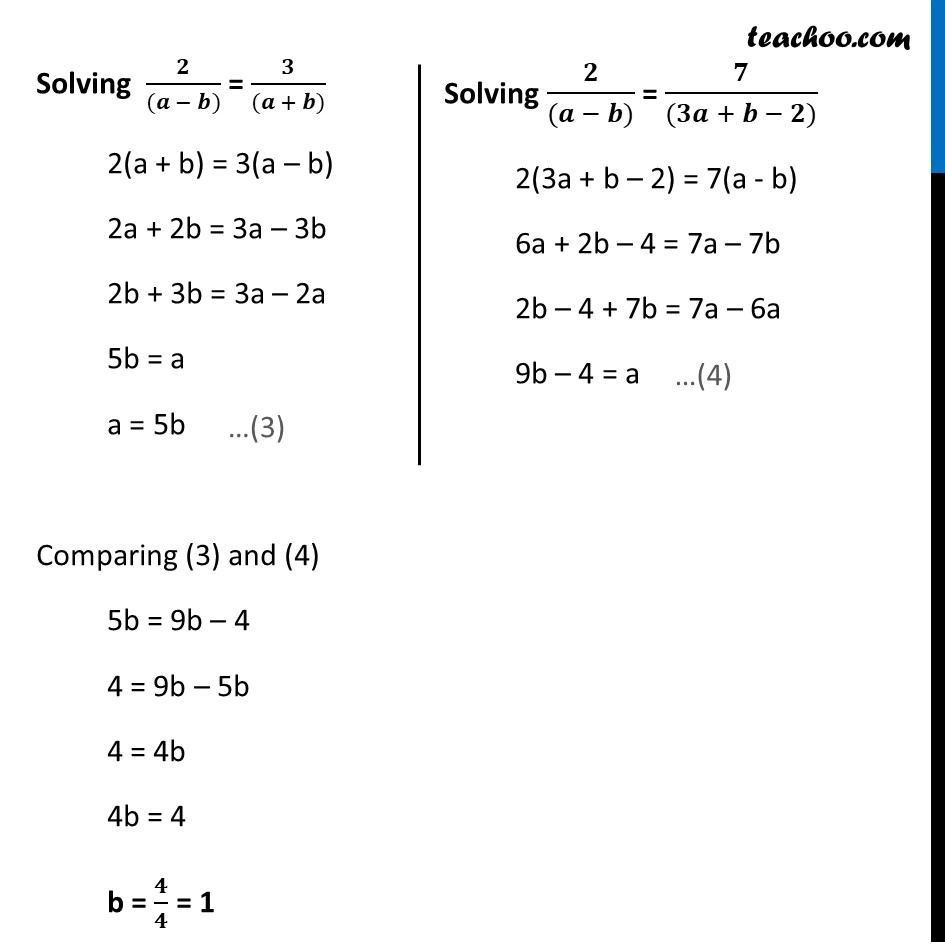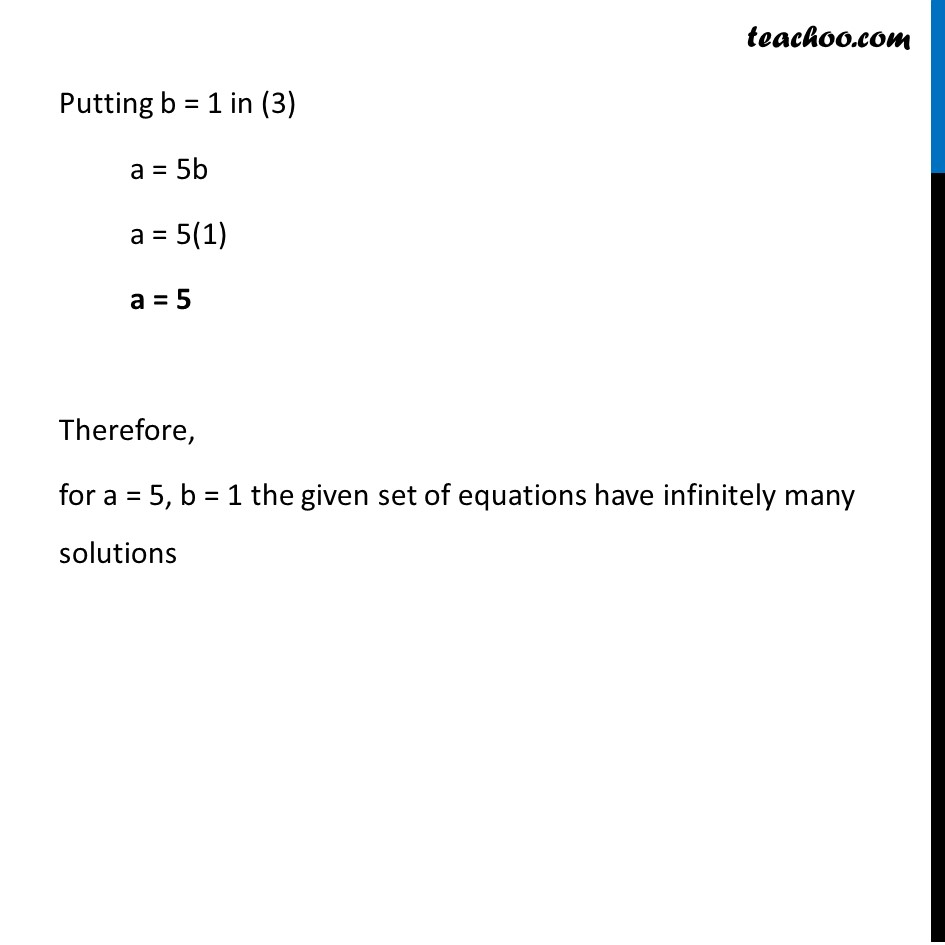Chapter 3 Class 10 Pair of Linear Equations in Two Variables

Class 10
Important Questions for Exam - Class 10Learn in your speed, with individual attention - Teachoo Maths 1-on-1 Class

### Transcript

Question 2 (i) For which values of a and b does the following pair of linear equations have an infinite number of solutions? 2x+ 3y = 7 (a – b) x + (a + b) y= 3a + b – 2 2x + 3y = 7 (a – b)x + (a + b) y = 3a + b – 2 2x + 3y = 7 2x + 3y – 7 = 0 Comparing with a1x + b1y + c1 = 0 ∴ a1 = 2,b1 = 3,c1 = –7 (a – b)x + (a + b)y = 3a + b – 2 (a – b)x + (a + b)y – (3a + b – 2)= 0 Comparing with a2x + b2y + c2 = 0 ∴ a2 = (a – b),b2 = (a + b), c2 = – (3a + b – 2) So, a1 = 2, b1 = 3, c1 = –7 & a2 = (a – b), b2 = (a + b), c2 = – (3a + b – 2) It is given that the equation has infinite number of solutions So, 𝒂𝟏/𝒂𝟐 = 𝒃𝟏/𝒃𝟐 = 𝒄𝟏/𝒄𝟐 Putting in values 2/((𝑎 − 𝑏)) = 3/((𝑎 + 𝑏)) = (−7)/(−(3𝑎 + 𝑏 − 2)) 𝟐/((𝒂 − 𝒃)) = 𝟑/((𝒂 + 𝒃)) = (−𝟕)/(−(𝟑𝒂 + 𝒃 − 𝟐)) Solving 𝟐/((𝒂 − 𝒃)) = 𝟑/((𝒂 + 𝒃)) 2(a + b) = 3(a – b) 2a + 2b = 3a – 3b 2b + 3b = 3a – 2a 5b = a a = 5b Solving 𝟐/((𝒂 − 𝒃)) = 𝟕/((𝟑𝒂 + 𝒃 − 𝟐)) 2(3a + b – 2) = 7(a - b) 6a + 2b – 4 = 7a – 7b 2b – 4 + 7b = 7a – 6a 9b – 4 = a Comparing (3) and (4) 5b = 9b – 4 4 = 9b – 5b 4 = 4b 4b = 4 b = 𝟒/𝟒 = 1 Putting b = 1 in (3) a = 5b a = 5(1) a = 5 Therefore, for a = 5, b = 1 the given set of equations have infinitely many solutions